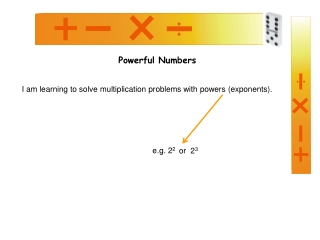DownloadDownload PresentationPowerful Numbers

# Powerful Numbers

Download Presentation## Powerful Numbers

- - - - - - - - - - - - - - - - - - - - - - - - - - - E N D - - - - - - - - - - - - - - - - - - - - - - - - - - -
##### Presentation Transcript

1. Powerful Numbers I am learning to solve multiplication problems with powers (exponents). e.g. 22 or 23

2. The square below is 4 cubes high and 4 cubes wide. The square below is 3 cubes high and 3 cubes wide. The square below is 2 cubes high and 2 cubes wide. We can work out how many cubes there are by working out 3 X 3 = 9 We can work out how many cubes there are by working out 2 X 2 = 4 We can work out how many cubes there are by working out 4 X 4 = 16 Using exponents we can record this as 32 Using exponents we can record this as 22 Using exponents we can record this as 42 If I square root the 4. If I square root the 9. If I square root the 16. I get 2. This is the length of the sides. I get 3. This is the length of the sides. I get 2. This is the length of the sides.

3. 43 Can be represented by a cube that is 4 small cubes high, wide and deep. 33 Can be represented by a cube that is 3 small cubes high, wide and deep. We can work out how many small cubes were used to make these by calculating 43 We can work out how many small cubes were used to make these by calculating 33 43 = 4 X 4 X 4 33 = 3 X 3 X 3 4 X 4 = 16 16 X 4 = 64 3 X 3 = 9 9 X 3 = 27

4. Numbers can be raised to powers of 4, 5, 6 etc. These numbers get big very quickly. Lets look at a couple of examples. Try to work these out as we go. 2 X 2 = 22 4 2 X 2 X 2 = 8 23 16 2 X 2 X 2 X 2 = 24 2 X 2 X 2 X 2 X 2 = 32 25 26 2 X 2 X 2 X 2 X 2 X 2 = 64

5. Work out answers to the questions below. Click again when you have worked out all your answers. 1) 33 2) 22 3) 24 4) 34 5) 27 27 4 16 81 128 Need some more practise? Try Pg47 & 48 of Teacher Tools Multiplication and Division Book Numeracy resources